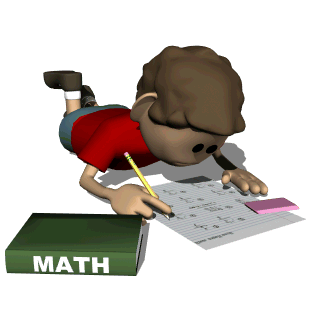• # Welcome to Grade 1 Math!## Focus

The major work of math in Grade 1 will focus on these areas:

• Addition and subtraction: representing these concepts with models like ten-frames and tape diagrams, and solving both word and calculation problems.
• Beginning to work with the properties of addition and subtraction, and understanding the relationship between these two concepts.
• Adding and subtracting within 20, with fluency expected within 10.
• Beginning to work with addition and subtraction equations, which includes the understanding that the equal sign means both sides of the equation have the same value.
• Understanding and using place value concepts, models and the properties of operations to add and subtract.
• Measure lengths by using objects, not rulers. (Rulers are introduced in second grade in connection with understanding the number line, halves, etc.)

Some additional work will be in these areas:

• Telling and writing time to the nearest half hour.
• Reasoning with shapes and their attributes.

We want our students to be great problem solvers and have many strategies to solve math problems. In class, the children will learn a variety of instructional strategies that will become foundational for work from grades 1 - 5. Resources, including video links, will be added to this page to help you assist your child at home.

"Procedural fluency is the ability to apply procedures accurately, efficiently, and flexibly; to transfer procedures to different problems and contexts; to build or modify procedures from other procedures; and to recognize when one strategy or procedure is more appropriate to apply than another." National Council of Teachers of Mathematics

By the end of Grade 1 , the goal is for students to be fluent with addition and subtraction within 10. Parents can help children memorize and work on these facts in a variety of ways, like playing cards or other games with your children that have to do with addition or subtraction. Fluency in this area is an important step for Grade 2.

## Units of Study

Eureka Math is comprised of units called Modules. The modules build on each other, creating a unified sequence of topics to help build student understanding and the development of computation skills and the ability to reason mathematically. A lot of time is spent on building conceptual understanding, meaning we spend a lot of time with models such as drawings and objects, in order to build a strong foundation of understanding how math works, not just how to solve quick addition or subtraction equations.

Module 1: Sums & Differences to 10
Module 2: Introduction to Place Value Through Addition & Subtraction Within 20
Module 3: Measurement - Ordering and Comparing Length Measurements as Numbers
Module 4: Place Value Comparison: Addition & Subtraction to 40
Module 5: Identifying, Composing & Partitioning Shapes
Module 6: Place Value Comparison: Addition & Subtraction to 100